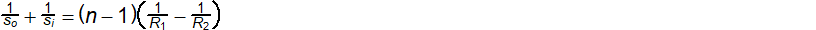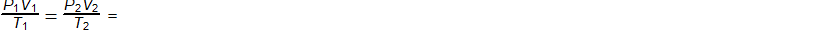### AP Physics 1 Week 5 (July 9 - July 15, 2017)

Part 1:  Algebra (Rearrange)

1.  solve for n2.  solve for si3.  solve for R14.  Solve for T15.  Solve for V2Part 2:  Algebra (Inverse and Direct Relationships)

6. The work done in stretching a spring is directly proportional to the square of the elongation.  If 12 foot-pounds of work are done in stretching a spring 2 feet beyond its natural length of 10 feet, find the work done in stretching it to be a length of 13 feet.  (DBW, p224, #11)

7.  The amount of silt carried by a stream is directly proportional to the sixth power of its velocity.  A certain stream carries 150 tons of silt per day.  Determine the amount of silt it would carry it its velocity were doubled.  (DBW, p224, #12)

8.  The frequency of a radio wave is inversely proportional to the wave length.  If a wave 250 meters long has a frequency of 1200 kilocycles per second, find the length of a wave with a frequency of 800 kilocycles. (DBW, p316, #1)

9.  A pulley with a diameter 8 inches is running at 1452 revolutions per minute while belted to a 12 inch pulley.  Find the speed of the larger pulley if the speeds of the pulleys are in inverse proportion to their diameters.  (DBW, p316, #2)

10.  Two meshed gears have 35 and 36 teeth respectively.  If the speeds are inversely proportional to the numbers of teeth, at what speed should the second gear be driven so that the first gear will run at 1450 revolutions per minute?  (DBW, p316, #3)

Part 3: Geometry/Trigonometry
(For Physics, make sure your calculator is set in degrees)

For angle measurements in physics and astronomy, degrees, whether as decimal degrees or in the form of degrees, arcminutes and arcseconds are used for describing the direction of motion or implied force.  When discussing circular motion, there may be a need to state an answer in terms of radians or even revolutions (rotations).  In general. 1 revolution or rotation of a circle about an axis is equal to 2p radians or 360o.  This is a relationship you were introduced to when you had to first learn the unit circle.

Surveyors use gradians, a way of decimalizing the unit circle based on units of ten, whereas, each of the 4 quadrants is set at a constant measure of 100 gradians.  Thus, 0o is 0 gradians, 90o is 100 gradians, 180o is 200 gradians, 270o is 300 gradians, and 360o is 400 gradians.  Thus, a gradian is a measure that is equal to 1/400 of a turn or rotation.

A mil is used in military science, defined as 1/6400 of the circumference of the circle, thus, it is approximately 1/1000 of a radian.  Since 6400 mils is equal to 360o, then 1 mil = (9/160)o, or 1o = (160/9) mil.  We will not use gradians or mils in this class, this exercise is just to force you to think about performing simple conversions in units you do not normally use.

11.  30o

12.  135o

13.  255o

14.  25o 30'

15.  42o 24' 35"

Part 4:  Word Problems

16.  Two railroad workers are together in a 0.6 mile mountain tunnel.  One walks east

and the other walks west in order to be out of the tunnel before the "express"

train comes through at 30 mph.  Each man reaches his respective end of the

tunnel in 3 minutes.  If the man walking east reaches the east entrance just as

the train enters, and the train passes the other man 0.12 miles beyond the

opening of the west end of the tunnel, at what speed did each man walk?

(DBW, p104, #29)

17.  Nancy and William start at the same time from a mailbox.  Nancy travels north

while William travels west.  Williams speed is 4 mph greater than twice that of

Nancy's.  After one hour, they are 26 miles apart.  What is the speed of each

person? (DBW, p138, #18)

18.  After making a trip of 126 miles, a lady found that if she had increased her average speed by 8 mph, she could have made the trip in 1 hour less time.  Find her

original speed. (DBW, p181, #4)

19.  A rowing crew times itself on a 3 mile course.  The 3 miles upstream take 20

minutes, and the 3 miles downstream takes 12 minutes.  What is the speed of

the rowing crew in still water? (DBW, p182, #13)

20.  With the wind behind him, a skater crossed a frozen river 1 mile wide in 3 minutes.              He skated straight into the wind on his return trip, which took 15 minutes.  Find

the speed of the wind. (DBW, p182, #14)